# Catch up on the latest AI articles# RELIC: A Causal Interpretation Of Contrastive Learning At Large.

3 main points
✔️ A paper that tries to interpret contrastive learning from a causal perspective
✔️ Proposed RELIC Loss with a restriction that the style of the image does not affect the task
✔️ Verified that the learned representation is robust and generalizable on ImageNet-C and ImageNet-R

written by Jovana MitrovicBrian McWilliamsJacob WalkerLars BuesingCharles Blundell
(Submitted on 15 Oct 2020)

Subjects: Machine Learning (cs.LG); Computer Vision and Pattern Recognition (cs.CV); Machine Learning (stat.ML)code：The images used in this article are from the paper or created based on it.

## first of all

Contrastive Learning ( CCL ) is currently playing a big role, including SimCLR(Commentary Article ) and BYOL (Commentary Article ). Because it is self-supervised learning and does not require label data, it can be pre-trained on huge amounts of data and applied to downstream tasks, such as BERT, and is attracting a lot of attention.

On the other hand, the theoretical interpretation of the principle of contrast learning has not been completed yet. At one time, the theory that mutual information maximization between an image and its representation is important was a popular one, but the paper On Mutual Information Maximization for Representation(commentary article ) showed that mutual information theory alone cannot interpret contrast learning.

The theory has not been able to keep up with the unprecedented and booming development of contrast learning. Today I'd like to share a paper published by DeepMind that provides a theoretical interpretation of contrast learning that incorporates the idea of causality.

2. proposed method: REpresentation learning via Invariant Causal mechanisms (RELIC)

The authors proposed a. a causal interpretation of previous studies on contrastive learning and b. a RELIC loss function. We will explain them in turn.

### 2. Interpreting prior research on contrastive learning with a causal theory

The causal graph drawn by the authors consists of a Data generation part and a Representation Learning part.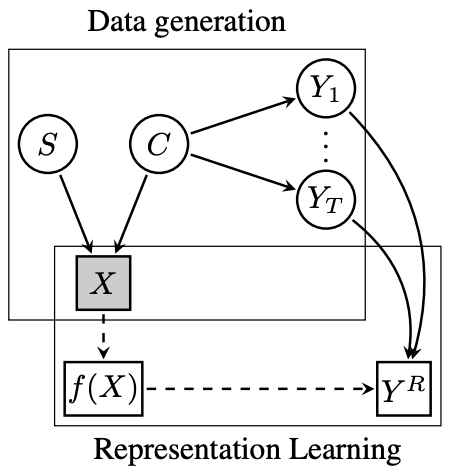Data generation includes the following three hypotheses

1. Image (X) is generated from content (C) and style (S)
2. Only the content (C) affects the downstream tasks (Y_1... .Y_T).
3. Content (C) and style (S) are independent of each other

Representation Learning solves a proxy task (Y^R) by learning an f(X) representation from an image (X). The surrogate task (Y^R) here is solved from the downstream tasks (Y_1.... . Y_T). In other words, the downstream task (Y_1. . Y_T). In other words, we assume that the proxy task (Y^R) must be able to learn enough information to solve the downstream task (Y_1.

Here, the instance classification task, which is performed in existing Contrastive Learning, is a proxy. In contrast to the downstream task of classification, the instance classification task is more difficult because it is a task to distinguish an image itself from other images. Therefore, the downstream task can theoretically be solved by learning a representation f(X) that can solve this more difficult proxy task. This may be the reason why existing contrast learning works so well.

### 2. b Proposed RELIC loss function

We can now interpret the prior work on contrastive learning using a causal graph (the formula is shown later). The authors of this study assume that one of the elements of the image (X), style (S), does not affect the downstream task, so they restrict equation (2) that changing style (S) when solving the proxy task does not change the result.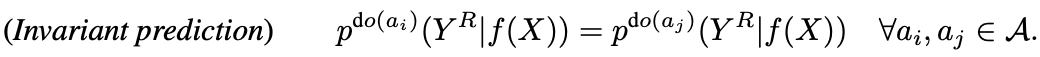That is, we adapt two different extensions from the set of data extensions A to the image so that the result in the proxy task (Y^R) is invariant. If we rewrite it as a loss function, it looks like this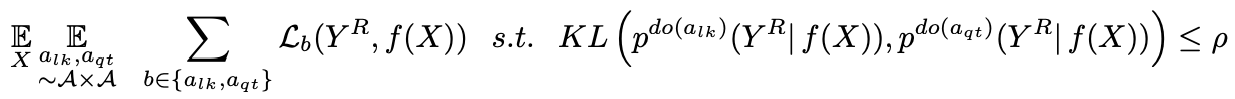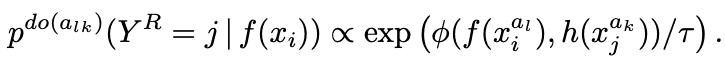Combining this loss function with the loss function used in traditional contrast learning yields equation (3).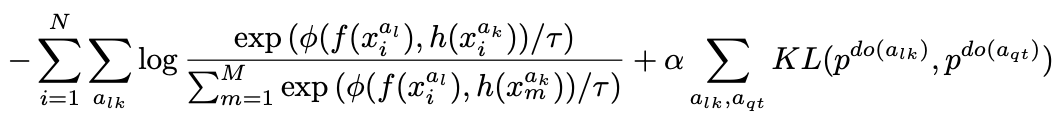It can be seen by referring to the following table that the loss function used in the traditional contrast study can be represented by the first entry in equation (3).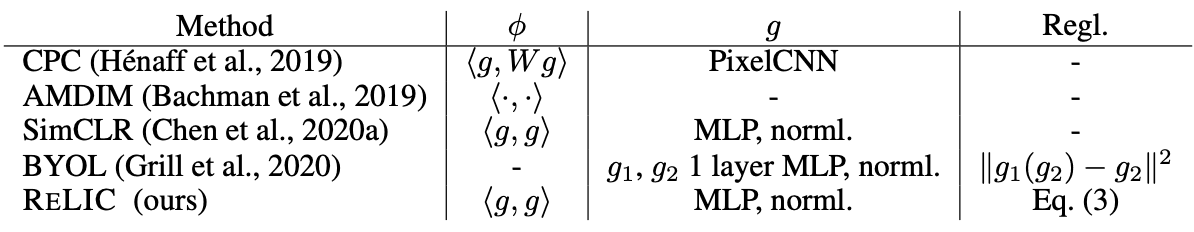The notation of the functions in Equation 3 and the table is explained: f() is a function that learns a representation from data, and h() is a function with the same architecture as f(). However, when using a moving average of the parameters of f() without updating the parameters of h() itself, it is called a target network architecture. Also, g() is used to map the expressions obtained by f() and h() to smaller latent variables, and Φ() is used to measure the distance between the latent variables mapped by g().

Therefore, the proposed RELIC loss function (Equation 3) combines the traditional loss function with the restriction that style (S) should not affect the proxy task.

The following figure illustrates the proposed RELIC loss function. It can be interpreted that the similarity probability distributions of two representations obtained by two different transformations of the input are close to each other by KL distance.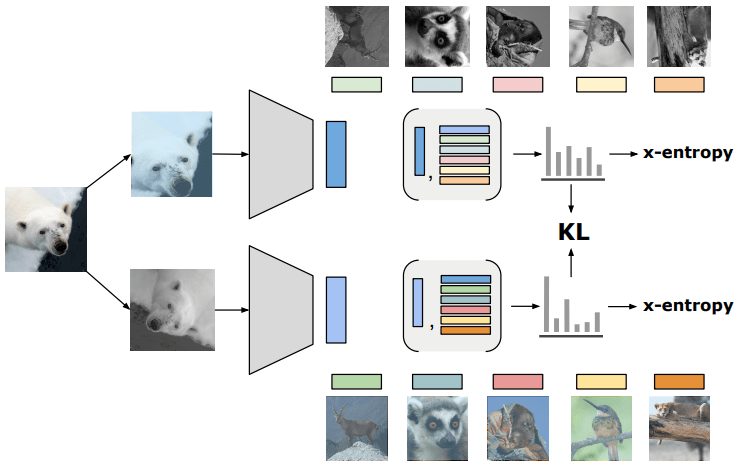This is the end of the introduction of the proposed method, but since the highlight of this work is the theoretical proof of these causal restrictions and interpretations, it may be helpful to refer to the proof in Appendix B.

## experiment

In the experimental part, we a. linearly evaluate the goodness of the learned representations; b. evaluate the robustness and generalizability of the representations obtained by the proposed method by removing the effect of style(S); c. evaluate the robustness and generalizability of the representations obtained by the proposed method by removing the effect of style(S).

### 3. an Evaluating the quality of representation with linear classification

Fischer's linear discriminant ratio. Fischer's linear discriminant ratio.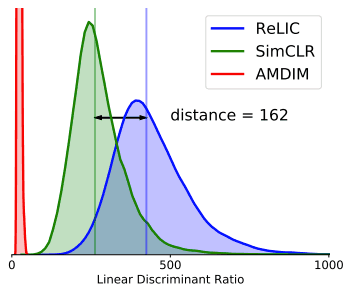First, we use the ease of linear classification as a measure to evaluate the quality of the learned representation. The metric used here is Fischer's linear discriminant ratio, which is calculated by the following equation.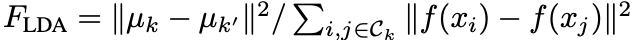However, it is at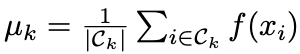.

The larger this value, the better the representation, so we can see that it is a good representation compared to Baseline's SimCLR.

・ImageNet

Next, we perform a linear evaluation using ImageNet.

The problem setting for contrastive learning is representation learning without supervised labels using ImageNet. The classification accuracy is measured by attaching a new linear classifier to the obtained representation. Since a simple linear classifier is used, it is thought that the goodness of the representation directly contributes to the classification accuracy, and it is a standard evaluation method.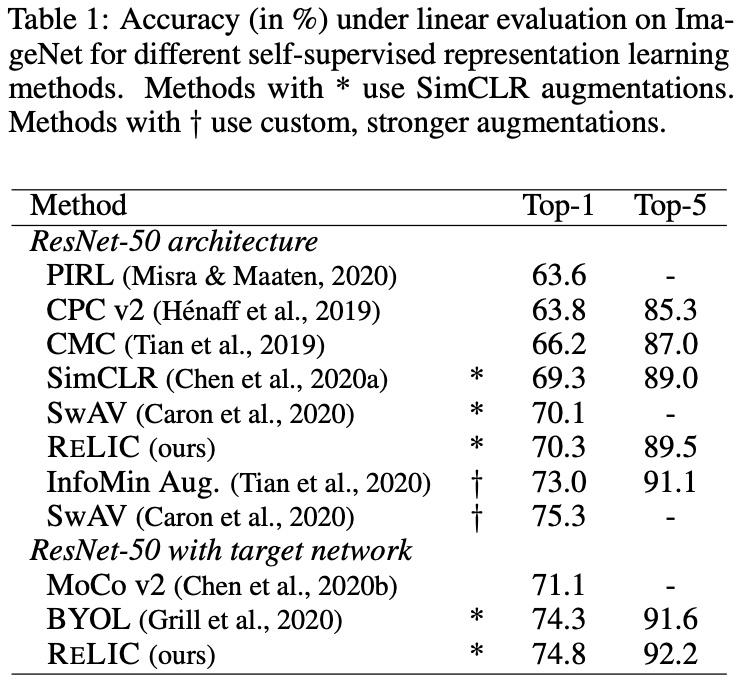The proposed method RELIC is available in two versions, SimCLR based and BYOL based (with Target Network). The above figures show the results of each of them and they are comparable to the previous studies. However, the SimCLR-based RELIC without Target Network is not as good as methods such as InfoMin Aug. and SwAV, which the authors attribute to the use of more powerful data extensions in the comparison method. This result shows that data expansion plays an important role in contrast learning.

### 3. b Evaluating the robustness and generalizability of RELIC on different data sets

Evaluate robustness with ImageNet extended dataset

Although the linear evaluation using ImageNet did not show any remarkable results, we evaluated the proposed method RELIC for robustness because it is considered to be a representation that learns only the information of content (C), removing the influence by style (S).

We first measure the classification accuracy using the ImageNet-R dataset (Figure 7.), which is different from the pre-training dataset.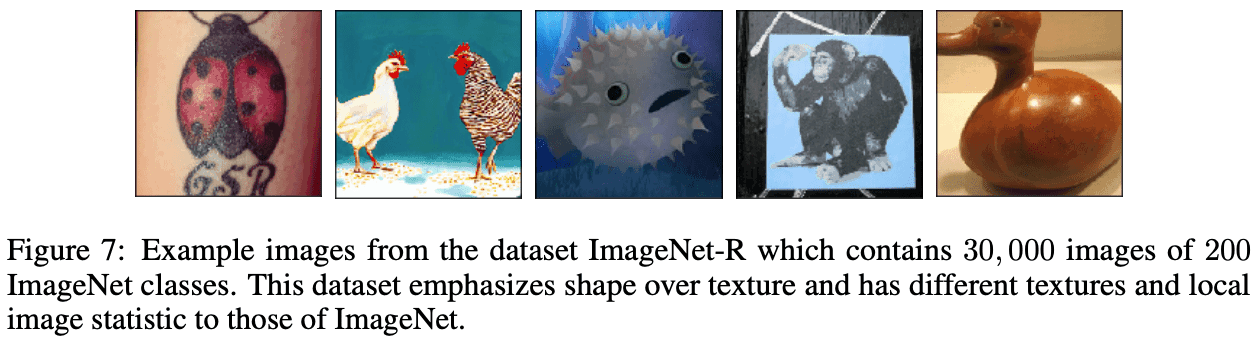Table 2. shows the results of the comparison between supervised learning, SimCLR, and BYOL using the error rate of classification as a measure. We can see that the accuracy is worse than supervised learning but better than the comparison methods.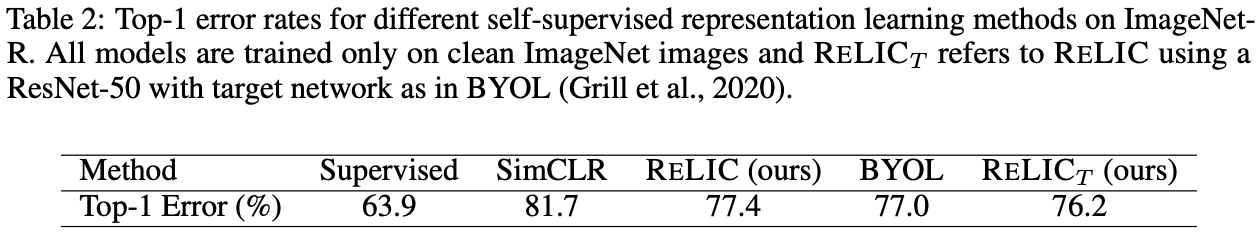In addition, we evaluated the robustness of the dataset ImageNet-C, which consists of 75 different interferences on the ImageNet images with 15 different noises (Fig. 5.) and different degrees of each (Fig. 6.).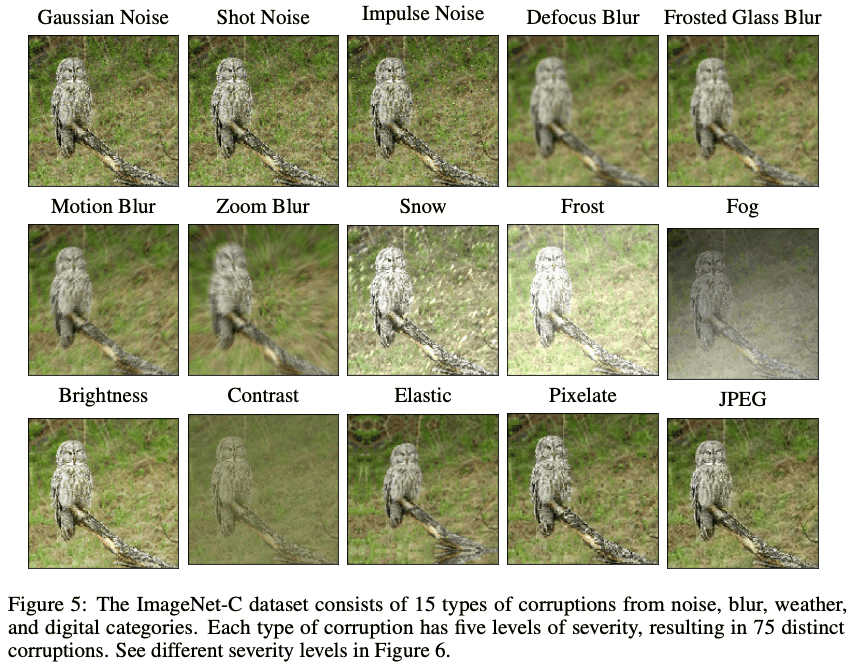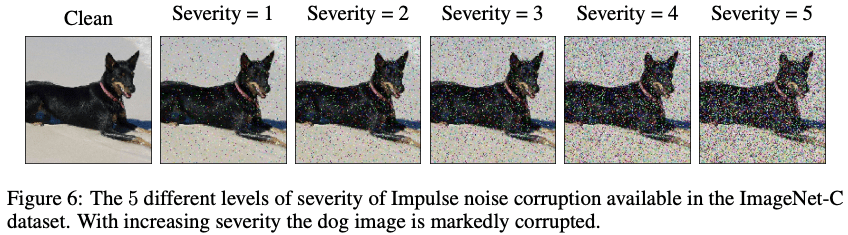Comparing several error rates as indices (Table 3.), we can say that the proposed method RELIC is indeed more robust. This shows the effectiveness of the loss function of the proposed method RELIC, which is intended to be invariant to style.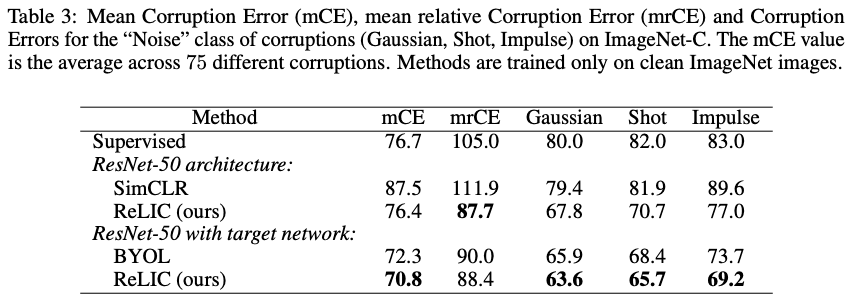Evaluation of generalizability in 57 Atari Games of reinforcement learning

One of the attractive features of representation learning is that the good representations learned can be applied to downstream tasks. In this work, the representations obtained by the proposed method RELIC are evaluated and experimented on the reinforcement learning benchmark 57 Atari Games, which is based on R2D2, a method that combines RNN and reinforcement learning DQN, and compared with CURL and BYOL.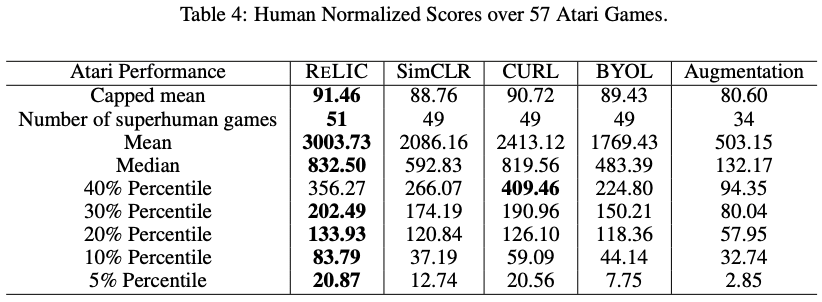The results presented in Table 4. show that the proposed method has better generalizability than SimCLR and BYOL. We also compare our results with the work proposed in a reinforcement learning study called CURL. I think that representation learning rarely experiments with reinforcement learning benchmarks, so I found this to be an interesting experiment that is unique to DeepMind.

## summary

This article presented an integrative interpretation of the research on contrastive learning. It was a theoretical approach that is unique to causal theory, with a theorem proving that is too rigorous to be written in an article. If you are interested in learning more about the details, and if you are confident in your mathematics, I encourage you to open the paper and read it.

In a nutshell, the study was implemented using the RELIC loss function defined under the assumption that the style (S) does not affect the task.

On the other hand, a similar study that focuses on data augmentation for controlled learning and argues on a causal basis that style(s) and content(s) should be separated is the June 2021 Self-Supervised Learning with Data Augmentations ProvablyIsolates Content from Style.

As you can see, many related studies are still being published, and we will continue to pay attention to this very interesting field.

If you have any suggestions for improvement of the content of the article,